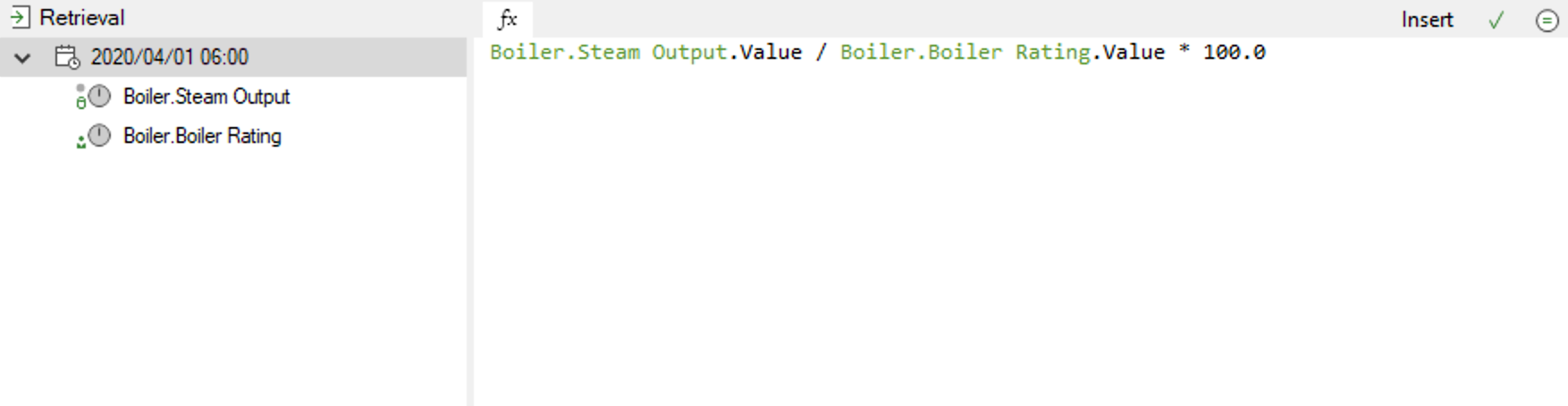Follow

# What is a Calculated Measure?

Calculated Measures are used to configure your own calculations based on one or more measures in your Flow System. This is an extremely powerful feature which allows you to generate additional information, just like you would be able to do in Excel. For example, if you had an hourly measure for production counts, and an hourly measure for electricity used, you could create an hourly calculated measure for the ratio of electricity used per unit of production.

In addition to allowing the configuration of calculations, Flow has a built-in library of common functions, similar to what you would find in Excel. You can even create your own User Defined Functions to standardize and simplify your calculation expressions.

Flow allows you to use Calculated Measures inside other Calculated Measures, making nested calculations possible.

It is possible to build complex calculations using Calculation Extensions in Flow.

To configure a Calculated Measure, change the Retrieval Type combobox in the Retrieval section of the Measure Editor to "Calculated from other Measures".  Alternatively, a quick way to create a Calculated Measure is to drag a measure onto the calculator icon in the Flow Zone.

Notice the Retrieval section properties for a Calculated Measure:For a Calculated Measure, the “Retrieval” tab displays two sections:

 Dependants The calculation dependants are displayed on the left.  Calculations in Flow are defined by a Date and Time from which the calculation is valid.  Additional calculations can be added to the dependency tree, as long as they have a different Date and Time.  This mechanism provides the ability to change calculations over time, but not lose the ability to backfill a measure to a time when a different calculation was used Expression The expression on the right is the actual calculation, which is defined using Microsoft.NET’s C# syntax.  Flow simplifies the “writing” of the expression by supporting double-click and drag ‘n drop into the expression

Notice that the calculation expression makes use of the “.Value” property of a measure.  The “Insert” button allows you to use other properties of a measure (i.e. “.Quality”, “.Duration” in milliseconds, “.PeriodStart” and “.PeriodEnd”)
Calculation “Date and Time"
You will notice in the calculation dependency tree that a calculation’s dependents and expression is defined by a date and time.  This represents the date and time from which this calculation is valid. This functionality allows for calculations to change over time (i.e. water reticulation calculation changes because of a new meter installation), but still maintain data integrity during a measure “backfill”.